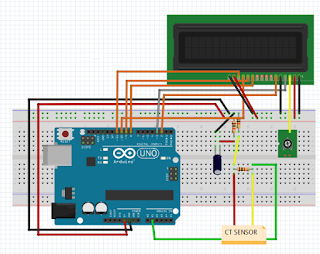# Measure Electric Current Sensors Using CT and Arduino

In the electric power system there is a piece of equipment known as CT. What then is the CT it? CT is an abbreviation of Current (current) Transformer (changer). As the name implies, CT is an equipment that converts the current magnitude from large to small or otherwise in accordance with the necessary requirements.

For power systems are needed CT power to change the nominal value of the current system becomes smaller so that it can be read by equipment protection or measurement (metering). Protection and metering equipment typically only receive the current value of the two nominal values ​​are 0-1A (for first-class equipment 1A) and 0-5A (for first-class equipment 5A).

Protection and metering equipment will only read the output value of CT (from the CT secondary terminals) and then calculate / change it back as the reading of the primary side (the actual value of the current flowing). Value calculations performed by the protection and metering equipment is based on the value of a CT ratio.

### Selection Ratio CT

To determine the value of CT to be used, a designer must know the value of the full load of the system of generation, transmission and distribution. As an example:

There is a power plant with a nominal 150kV 60MVA, then the value of CT is used?

note: Un = 150.000V, Pn = 60.000.000VA,

then In = Pn / Un = 60.000VA / 150000V

and the result is 400A (for the CT primary value)

If the nominal value of the primary current CT (fullscale) is already known, the next step is the selection of the nominal value of the secondary current CT. CT secondary current nominal value must be adjusted to the class equipment to be used. If the equipment using the input current of 5A class then selected CT ratio is 400 / 5A, as well as for equipment with input current 1A class, the CT ratio is 400 / 1A.

On CT with a ratio of 400 / 5A mean CT must issue a current value of + 5A on the secondary side when the CT primary side energized by + 400A (small large primary voltage does not affect the current CT). Then if in the secondary terminal CT measured current of 3.26A, what is the real value of the CT primary?

replied:

note: Ip / Is = 400 / 5A, Is (actual) = 3.26

then the value Ip (actual) = 3.26 x (400/5)

and the result is Ip (actual) = 260.8A

#### Accuracy Ratio CT

Each CT has an accuracy class reading error (% error) different. The smaller the value of reading error (% error) CT, the better the accuracy of a CT class. If there is new from the manufacturer with a CT ratio of 400/5 specifications and accuracy class 0.5. So what is the permissible values ​​that CT can be used?

replied:

According to IEC standard, that the current injection for testing the ratio of CT may be made from 10% (considering the scarcity of test equipment that has a value of output current is large, if any would certainly complicate the process of installation of test equipment in terms of weight, the cable used, size, etc.). So here, the primary side current CT can be injected worth 40A (minimum).

note: Ip / Is = 400/5, Ip (act.) = 40A

then Is = 40A x (400 / 5A)

and the result is = 0.5A, for a value of error of 0.5%, the current measurement results on the secondary CT terminal should not exceed 0.5025A or less than 0.4975A.

#### Schematic CT-Sensor and Arduino as follows:## Schematic CT-Sensor and Arduino

Coding :

```// Free Tutorial Arduino
// www.ioisalman.com

#include "EmonLib.h"                   // Include Emon Library
EnergyMonitor emon1;                   // Create an instance
#include
LiquidCrystal lcd(11, 10, 5, 4, 3, 2);

void setup()
{
Serial.begin(9600);

emon1.current(1, 111.1);             // Current: input pin, calibration.
lcd.begin(16,2);
lcd.setCursor(0,0);
lcd.print(" The electricity flow is: ");

}

void loop()
{
double Irms = emon1.calcIrms(1480);  // Calculate Irms only

Serial.print(Irms*230.0);         // Apparent power
Serial.print(" ");
Serial.println(Irms);          // Irms

lcd.begin(16,2);
lcd.setCursor(0,1);
lcd.print("    ");
lcd.setCursor(0,1);
lcd.print(Irms);

}
```

1.